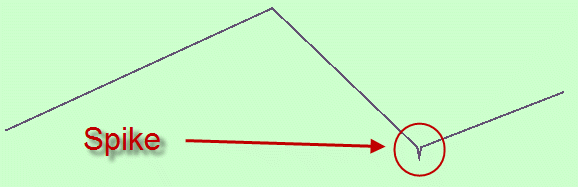# A simple spike finder for Spatial

This is an old article that uses older custom code e.g. Vector2Geom(). Because the principles in the code are correct, I will bring the code up to date if anyone shows any interest.

When investigating geometric or topological errors for linear or polygonal geometry objects, a range of tools are needed. Oracle has basic tools which can be used as the building blocks for additional tests. These are:

One test that is not clearly provided for is the discovery of “spikes” in the linestring defining an outer or inner ring of a polygon (or any linestring).

Dictionary definitions of a spike include:

noun
1. a nail-like fastener, 3 to 12 inches (7.6 to 30.5 cm) long and proportionately thicker than a common nail, for fastening together heavy timbers or railroad track.
2. something resembling such a nail; a stiff, sharp-pointed piece or part: to set spikes in the top of a cement wall.
3. a sharp-pointed piece of metal set with the point outward, as on a weapon.
4. an abrupt increase or rise: a chart showing a spike of unusual activity in the stock market; a sudden spike of electrical current.
5. a rectangular or nail-like metal projection on the heel and sole of a shoe for improving traction, as of a baseball player or a runner.

9. a pointed portion of a continuous curve or graph, usually rising above the adjacent portion: a spike in the value of the voltage.

Applying these to geographic/geometric linear objects we could say that a “spike” is:

is a sharp, abrupt and narrow deviation from the normal course of a linestring

Graphically:How can we detect spikes?

One simple (the point of this article) method for finding a spike is to see if the gap between the two “vectors” that compose the spike collapse at the specified tolerance of a table’s geometry column (user_sdo_geom_metadata) causing a topological error (often 13349). Here are two examples of spikes.

A simple algorithm for finding spikes is to walk all the adjacent “vectors” of a linestring and send each pair into SDO_GEOM.RELATE with a “determine” mask. If the result is anything other than TOUCH we must have an error.

```    /** ----------------------------------------------------------------------------------------
* @function   : FindSpikes
* @precis     : Function that implements a simple "spike" finder.
* @version    : 1.0
* @usage      : SELECT b.* FROM test a, TABLE(FindSpikes(a.geom,0.005) b;
* @param      : p_geometry  : MDSYS.SDO_GEOMETRY : An sdo_geometry line or polygon object.
* @param      : p_tolerance : NUMBER             : Oracle sdo_tolerance value eg 0.005 meters for geodetic.
* @history    : Simon Greener - October 2011 - Original coding.
**/
CREATE OR REPLACE
FUNCTION FindSpikes(p_geometry    IN mdsys.sdo_geometry,
p_tolerance   IN NUMBER DEFAULT 0.005)
RETURN CODESYS.SDO_ERROR.T_VectorSet pipelined
AS
v_vector       CODESYS.t_vector;
v_vectors      CODESYS.SDO_ERROR.T_VectorSet;
v_num_elements pls_integer;
v_element_no   pls_integer;
v_element      mdsys.sdo_geometry;
v_num_rings    pls_integer;
v_ring_no      pls_integer;
v_ring         mdsys.sdo_geometry;
v_relate       varchar2(100);
NULL_GEOMETRY  EXCEPTION;
NOT_LINE_POLY  EXCEPTION;
BEGIN
IF ( p_geometry IS NULL ) THEN
Raise NULL_GEOMETRY;
ElsIf ( p_geometry.get_gtype() NOT IN (2,5,3,7) ) THEN
RAISE NOT_LINE_POLY;
END IF;
-- Process Line/Multiline and Polygon/Multipolygon
--
v_num_elements := MDSYS.SDO_UTIL.GETNUMELEM(p_geometry);
<<Process_All_Elements>>
FOR v_element_no IN 1..v_num_elements LOOP
v_element := MDSYS.SDO_UTIL.EXTRACT(p_geometry,v_element_no,0);
IF ( v_element IS NOT NULL ) THEN
-- Polygons
-- Need to check for inner rings
--
IF ( v_element.get_gtype() = 3) THEN
-- Process all rings in this single polygon have?
v_num_rings := GetNumRings(v_element);
<<All_Rings>>
FOR v_ring_no IN 1..v_num_rings LOOP
v_ring            := MDSYS.SDO_UTIL.EXTRACT(p_geometry,v_element_no,v_ring_no);
-- Now walk ring's vectors
--
IF ( v_ring IS NOT NULL ) THEN
-- Process 1st/2rd vectors looking for spike
SELECT CODESYS.T_Vector(v.id,V.Startcoord,V.Endcoord) AS vector
BULK COLLECT INTO V_Vectors
FROM TABLE(CODESYS.Sdo_Error.GetVector(v_ring)) V
ORDER BY v.id;
IF NOT ( V_Vectors IS NULL OR V_Vectors.COUNT = 0 ) THEN
FOR I IN V_Vectors.FIRST..V_Vectors.LAST Loop
IF ( i = V_Vectors.LAST ) THEN
v_vector := v_vectors(1);
ELSE
v_vector := v_vectors(i+1);
END IF;
-- Check if the interact in a way other than TOUCH
--
v_relate := mdsys.sdo_geom.relate(Vector2Geom(v_vectors(i),p_geometry.sdo_srid),
'DETERMINE',
Vector2Geom(v_vector,p_geometry.sdo_srid),p_tolerance);
IF ( v_relate <> 'TOUCH' ) THEN
PIPE ROW ( CODESYS.T_Vector(v_vectors(i).id,v_vectors(i).startCoord,v_vectors(i).endCoord)  );
PIPE ROW ( CODESYS.T_Vector(v_vector.id,v_vector.startCoord,v_vector.endCoord) );
END IF;
END Loop;
END IF;
END IF;
END LOOP All_Rings;
-- Linestrings
--
ElsIf ( v_element.get_gtype() = 2) THEN
-- Process 1st/2rd vectors looking for spike
SELECT CODESYS.T_Vector(v.id,V.Startcoord,V.Endcoord) AS vector
BULK COLLECT INTO V_Vectors
FROM TABLE(CODESYS.Sdo_Error.GetVector(v_element)) V
ORDER BY v.id;
IF NOT ( V_Vectors IS NULL OR V_Vectors.COUNT = 0 ) THEN
FOR I IN V_Vectors.FIRST..(V_Vectors.Last-1) Loop
v_vector := v_vectors(i+1);
-- Check if the interact in a way other than TOUCH
--
v_relate := mdsys.sdo_geom.relate(Vector2Geom(v_vectors(i),p_geometry.sdo_srid),
'DETERMINE',
Vector2Geom(v_vector,p_geometry.sdo_srid),p_tolerance);
IF ( v_relate <> 'TOUCH' ) THEN
PIPE ROW ( CODESYS.T_Vector(v_vectors(i).id,v_vectors(i).startCoord,v_vectors(i).endCoord)  );
PIPE ROW ( CODESYS.T_Vector(v_vector.id,v_vector.startCoord,v_vector.endCoord) );
END IF;
END Loop;
END IF;
END IF;
END IF;
END LOOP;
RETURN ;
EXCEPTION
WHEN NULL_GEOMETRY THEN
raise_application_error(c_i_null_geometry, c_s_null_geometry,TRUE);
RETURN;
WHEN NOT_LINE_POLY THEN
raise_application_error(c_i_not_line_poly,   c_s_not_line_poly,TRUE);
RETURN ;
END FindSpikes;
```

Applying this function to the two spikes above we get.

I hope this is of use to someone.